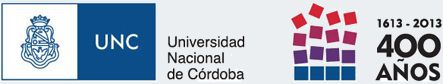# Statistical procedures

Infostat is a statistical analysis software that meets the needs of analysis of a wide range of users. The software has evolved rapidly and is frequently updated. These updates not only have to do with the addition of new functionality but also to increased productivity and algorithms' efficiency. InfoStat also evolve to keep the pulse of new hardware and operating systems, allowing us to maintain the code updated and adapted to the incredibly dynamic computer industry. InfoStat covers a wide range of statistical applications, below is a summary of the techniques implemented:

## Univariate

Exploratory Analysis
Construction of cross-classification tables
Construction of frequency tables and calculation of goodness of fit statistics
Calculate confidence intervals for parametric and nonparametric statistical sampling classic
Calculation of power and sample size for the design of experiment
Analysis of samples obtained under basic sampling designs
Inference in one and two populations using parametric and nonparametric methods

### Linear models for crossed and nested fixed factors (ANOVA)

Multiple comparisons (Fisher LSD, Duncan, Tukey, SNK, DGC, BSS, Scott & Knot, Bonferroni)
Contrasts

### Linear regression models

Simple
Multiple
Polynomial

#### Diagnostic Analysis

Residuals and predicted
Studentized residuals
Jackniffe residuals
Partial
residuals
Cook's distance
Leverage

Confidence and prediction bands

#### Model Selection

Forward
Backward
Stepwise
All possible models

### Nonlinear regression models

User-specified models
Logistics
Logistics to shift

4P-L (4-parameter logistic
5P-L (logistics 5 parameters)
Gompertz
Gompertz to shift
Exponential
Monomolecular
Richard
Linear installments
General linear and mixed models

Logistic
Poisson
Probit

### Correlation analysis

Pearson correlation coefficients, Spearman correlation,
Partial correlation coefficients
Path analysis

## Multivariate

### Reduction of dimensionality

Principal component analysis
Principal coordinates analysis (multidimensional scaling)
Biplots
Generalized Procrustes
Canonical correlations

PLS

### Discriminant Analysis

Linear discriminant
K nearest neighbors
Filtering attributes

### Cluster Analysis

#### Hierarchical

Centroid method
Weighted centroid method
Ward
Dendrograms and dendrograms

#### No hierarchical

Kmeans
Kmeans for large samples

### Special Applications

Dissimilarity and similarity matrix

### Epidemiology

Curves of sensitivity and specificity
Curves for positive and negative predictive value
ROC Curves
Survival curves of Kaplan-Meier

### Statistical for quality control

Control & chart attributes
Control variables diagrams

Pareto diagrams
Ability to process

### Didactic Applications

Graphics continuous density functions and probability graphing calculator
Confidence Intervals
All possible samples
Sample from the empirical distribution
Resampling

### Probabilities and quantiles calculator